Courses

# Test: Compounds of Halogens

## 25 Questions MCQ Test Chemistry Class 12 | Test: Compounds of Halogens

Description
This mock test of Test: Compounds of Halogens for JEE helps you for every JEE entrance exam. This contains 25 Multiple Choice Questions for JEE Test: Compounds of Halogens (mcq) to study with solutions a complete question bank. The solved questions answers in this Test: Compounds of Halogens quiz give you a good mix of easy questions and tough questions. JEE students definitely take this Test: Compounds of Halogens exercise for a better result in the exam. You can find other Test: Compounds of Halogens extra questions, long questions & short questions for JEE on EduRev as well by searching above.
QUESTION: 1

### Direction (Q. Nos. 1- 10) This section contains 10 multiple choice questions. Each question has four choices (a), (b), (c) and (d), out of which ONLY ONE is correct. Q.  Regarding the properties of hydrogen halides, which is wrong order?

Solution:

The correct order of % of ionic character is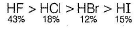QUESTION: 2

### The reaction 3ClO-(aq) → 2Cl- (aq) + ClO3-(aq) is an example of

Solution:

The reaction in which a species is simultaneously reduced and oxidised to form two different products is known as disproportionation reaction.

QUESTION: 3

### Which is incorrect statement?

Solution:

As we move down the group, stability of oxides decreases because the size increases.

QUESTION: 4

When gold and platinum dissolves in aqua-regia, the complexes formed are

Solution: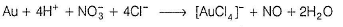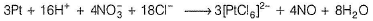QUESTION: 5

Mixture which contains equal amount of sodium chloride (NaCl) and sodium chlorate (NaClO3) gives off

Solution:

The correct answer is option A

On decomposition of NaClO3 , it gives NaCl and O in nascent form. it might get decomposed. As we know nascent [O] shows bleaching action due to its oxidizing properties.

QUESTION: 6

In perchloric acid (HCIO4), the number of σ and π-bonds

Solution:

The structure of perchlo ric acid (HCIO4) is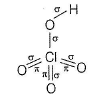5σ and 3π-bonds

QUESTION: 7

The correct order of acidic strength is

Solution:

The correct order of acidic strength is Cl2O7 > SO2 > P4O10

QUESTION: 8

Which of the following arrangements gives the correct order of increasing oxidation number of iodine?

Solution: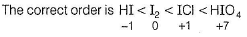QUESTION: 9

Which of the following is incorrect?

Solution: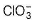has pyramidal while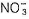has trigonal planar shape.

QUESTION: 10

Correct order of bond angles are in

Solution:

In CIO2, due to larger size of chlorine atom, repulsion between non-bonding electrons on the chlorine atoms overcome the lone pair-lone pair repulsion. Hence, the order of bond angle
CIO2 > Cl2O > H2O > OF2

*Multiple options can be correct
QUESTION: 11

Direction (Q. Nos. 11-15) This section contains 5 multiple choice questions. Each question has four choices (a), (b), (c) and (d), out of which ONE or MORE THAN ONE are correct.

Q.

Paramagnetic oxide among the following is/are

Solution:

Paramagnetic oxide has unpaired electrons or odd number of electrons.

QUESTION: 12

Which of the following shows disproportionation reaction?

Solution:

Cl2 + 2OH- → Cl + OCI- + H2O is a disproportionation reaction.

Cl0 → Cl-1

Cl0 → Cl+1

Hence correct option is B

QUESTION: 13

In chlorination of water, reactive oxygen atoms are produced by

Solution:
*Multiple options can be correct
QUESTION: 14

Which of the following reactions is/are not feasible?

Solution:

Iodine cannot displace Cl- and Br- from their salt and bromine cannot displace Cl-from their salt.

*Multiple options can be correct
QUESTION: 15

Bleaching powder liberate chlorine by

Solution: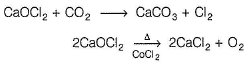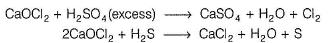QUESTION: 16

Direction (Q. Nos. 16 and 17) This section contains a paragraph, describing theory, experiments, data, etc. Two questions related to the paragraph have been given. Each question has only one correct answer among the four given options (a), (b), (c) and (d).

Passage

A salt A when heated with K2Cr2O7 and cone. H2SO4 liberates a gas which is absorbed in NaOH solution. The NaOH solution turns yellow. When this solution is acidified with acetic acid and lead acetate solution is added, a yellow precipitate B is formed. When A is mixed with MnO2 and heated with cone. H2SO4 , a gas C is evolved which turns a starch-iodide paper blue.

Q.

What is the colour of the gas which is evolved when salt A is heated with MnO2 and H2SO4?

Solution:

Greenish yellow gas is chlorine.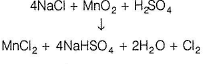QUESTION: 17

A salt A when heated with K2Cr2O7 and cone. H2SO4 liberates a gas which is absorbed in NaOH solution. The NaOH solution turns yellow. When this solution is acidified with acetic acid and lead acetate solution is added, a yellow precipitate B is formed. When A is mixed with MnO2 and heated with cone. H2SO4 , a gas C is evolved which turns a starch-iodide paper blue.

Q.

When the salt A is heated with cone. H2SO4, colourless gas is evolved which gives dense fumes when exposed with ammonia. The gas is

Solution:

Chloride salt produce colourless HCI vapours with conc. H2SO4 and these become dense due to NH4Cl on exposure to ammonia.

QUESTION: 18

Direction (Q. Nos. 18-20) Choices for the correct combination of elements from Column I and Column II are given as options (a), (b), (c) and (d) out of which one is correct.

Q.

Match the Column I with Column II and mark the correct option from the codes given below.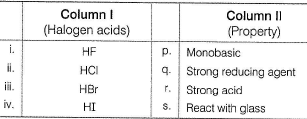Solution: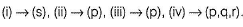QUESTION: 19

Match the Column I with Column II and mark the correct option from the codes given below.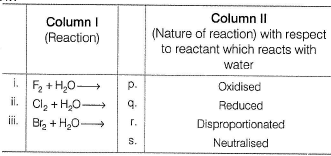Solution: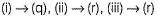QUESTION: 20

Match the Column I with Column II and mark the correct option from the codes given below.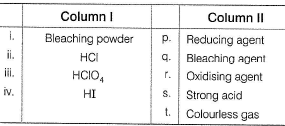Solution: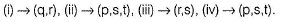*Answer can only contain numeric values
QUESTION: 21

Direction (Q. Nos. 21-24) This section contains 4 questions. When worked out will result in an integer from 0 to 9 (both inclusive).

Q.

The total number of lone pairs in Cl2O molecule are ............

Solution: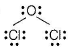Two lone pairs on oxygen and three lone pairs on each chlorine.

*Answer can only contain numeric values
QUESTION: 22

Number of sigma bonds present in Cl2O7 are .............

Solution: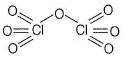*Answer can only contain numeric values
QUESTION: 23

Number of chemical species having sp3-hybridisation among these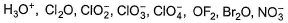Solution:

Except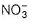, all others have sp3 hybridised atoms.

*Answer can only contain numeric values
QUESTION: 24

Aqua-regia is a mixture of one mole of cone. HNO3 and x moles of cone. HCI. Here, x value is ............

Solution:

Aqua-regia is a mixture of one mole of cone. HNO3 and 3 moles of cone. HCI.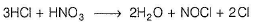QUESTION: 25

Direction (Q. No. 25) This section is based on Statement I and Statement II. Select the correct answer from the codes given below.

Q.

Statement I : On dehydration with P2O5 at 263 K, HCIO4 gives Cl2O7.

Statement II : This method is used in the preparation of Cl2O7

Solution:

On dehydration with P2O5 at - 10°C, HCIO4 gives Cl2O7 which is the anhydride of perchloric acid.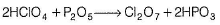This reaction has been used for the preparation of Cl2O7.